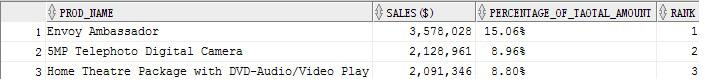# Oracle Reporting 1 - Ratio_to_Report Function

The RATIO_TO_REPORT function computes the ratio of a value to the sum of a set of values. RATIO_TO_REPORT ( expr ) OVER ( [query_partition_clause] ).
In this series of articles, I'm using Oracle's SH schema. It is modeled in star schema. The following query returns top 3 best-selling products in 2000, their sales amount, percentage of total amount, and the rank.

select * from (
SELECT prod.prod_name,
TO_CHAR(SUM(amount_sold),'9,999,999,999') "SALES(\$)",
to_char(RATIO_TO_REPORT(SUM(amount_sold)) OVER () * 100, '99.99' ) || '%' Percentage_of_Taotal_Amount,
rank() over(order by sum(amount_sold) desc) rank
FROM sales s, products prod
WHERE s.prod_id=prod.prod_id
AND trunc(s.time_id,'yyyy')=to_DATE('01-JAN-2000')
GROUP BY (prod.prod_name)
)
where rank <= 3;Ratio_to_report() Performance Analysis:
Consider this query:
WITH channel_summary AS (SELECT channels.channel_desc, SUM(amount_sold)
AS channel_total FROM sales, channels
WHERE sales.channel_id = channels.channel_id GROUP BY channels.channel_desc)
SELECT channel_desc, channel_total
FROM channel_summary WHERE channel_total > (SELECT SUM(channel_total) * 1/3
FROM channel_summary);

Statistics
----------------------------------------------------------
2  recursive calls
8  db block gets
1730  consistent gets
864  redo size
622  bytes sent via SQL*Net to client
523  bytes received via SQL*Net from client
2  SQL*Net roundtrips to/from client
0  sorts (memory)
0  sorts (disk)
1  rows processed
Oracle needs to use temp tablespace to save the data of with-clause, that's the reason of 2 recursive calls and 864 redo size. However, we can eliminate the writes to/reads from temp tablespace by using ratio_to_report.
Both queries run twice to avoid the effect of hard-parse.

select channel_desc, amount_sold from
(select ch.channel_desc, sum(s.amount_sold) amount_sold, ratio_to_report(sum(s.amount_sold)) over() ratio from channels ch, sales s where ch.channel_id=s.channel_id group by ch.channel_desc)
where ratio >= 0.33;

Statistics
----------------------------------------------------------
0  recursive calls
0  db block gets
1721  consistent gets
0  redo size
620  bytes sent via SQL*Net to client
523  bytes received via SQL*Net from client
2  SQL*Net roundtrips to/from client
1  sorts (memory)
0  sorts (disk)
1  rows processed

• 博文量
102
• 访问量
902136